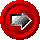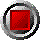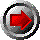European and American Equity Option FunctionsEuropean and American Equity Option Functions

The following functions are specialized to equity underlyings with discrete dividend payouts. Two option models have been implemented: the payout-adjusted European Black-Scholes (BS), and the control-variate American trinomial model (Trin).

Following Hull (see Technical Articles), we consider the stock price as the sum of two components: a stream of known, riskless dividends during the life of the option, and a risky ex-dividend component. For European options, the software subtracts the present value of dividends paid over the life of the option from the current stock price before applying the standard Black-Scholes model. Dividends are discounted using appropriate zero coupon rates, linearly interpolated from an array passed by the user. For American options, the present value of dividends is computed at each node in the lattice, taking into account of forward rates implicit in the zero curve.

There are four calling or "wrapper" functions comprising the set of equity option functions:

 EuroCallDivBS(argument list…) European call using the BS model EuroPutDivBS(argument list…) European put using the BS model AmerCallDivTrin(argument list…) American call using the Trin model AmerPutDivTrin(argument list…) American put using the Trin model

Each of the four wrapper functions allow the user to choose from seven different calculations, indexed by an alphabetic Output_Type code:

 P option price D delta (derivative with respect to price G gamma (second derivative with respect to price) V vega (derivative with respect to volatility, 1% change) T theta (time decay over next day) R rho (sensitivity to the riskless rate, per 1% change) I implied volatility

An Output_Type may be entered in either upper or lower case, and the user many substitute the entire word for the first letter if so desired (only the first letter is scanned).

The functions use the following arguments:

 Argument Description Restrictions Output_Type desired option calculation P, D, G, V, T, R, or I (see above) Valuation_Date option valuation date valid Excel date number Expiry_Date expiry date of option valid Excel date number >= Valuation_Date Stock_Price market stock price > 0 Strike strike price of the option > 0 Ex_Div_Dates array of stock ex-dividend dates strictly ascending order Dates less than or equal to Valuation_Date and greater than Expiry_Date are ignored Div_Amounts array of stock dividend amounts corresponding to Ex_Div_Dates array >= 0 Zero_Dates array of zero coupon curve dates strictly ascending order The first date of this array must be Valuation_Date Zero_Rates array of continuously compounded riskless rates in decimal form (e.g. six percent entered as 0.06) corresponding to Zero_Dates > 0 Tax_Factor factor to accommodate ex-dividend price drops different than the amounts in the Div_Amounts array, due to differential tax rates between dividends and capital gains typically be set to 1.0, or slightly less Vol, or Opt_Price underlying asset price volatility (risky portion of stock) in decimal form (e.g. twenty percent entered as 0.20), or market price of the option (when Output_Type = I) > 0 Tree_Steps extra argument used in trinomial model to specify number of tree steps in the lattice > 0 <= 300

© 1995-98 Leap of Faith Research Inc.